# Test : Factors And Multiples - 1

## 10 Questions MCQ Test Mathematics for Class 5: NCERT | Test : Factors And Multiples - 1

Description
Attempt Test : Factors And Multiples - 1 | 10 questions in 10 minutes | Mock test for Class 5 preparation | Free important questions MCQ to study Mathematics for Class 5: NCERT for Class 5 Exam | Download free PDF with solutions
QUESTION: 1

### Fraction is a _________

Solution:

fraction simply tells us how many parts of a whole we have. You can recognize a fraction by the slash that is written between the two numbers. We have a top number, the numerator, and a bottom number, the denominator. For example, 1/2 is a fraction.

QUESTION: 2

### Decimal comes from Latin word _________

Solution:

Decimal comes from the Latin word decimus, meaning tenth, from the root word decem, or 10.

QUESTION: 3

### Mixed fraction = Nearest Natural Number + _________

Solution:

Proper fraction

QUESTION: 4

Decimals having the same number of decimal places are called _________

Solution:

Like decimals

QUESTION: 5

The value of 0.25÷0.0025×0.025 of 2.5 is

Solution:

we have,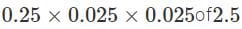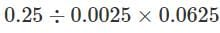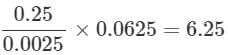QUESTION: 6

A cricket pitch is about 364 cm. In meters it is equal to

Solution: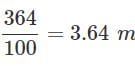QUESTION: 7

In the given figure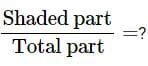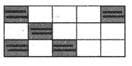Solution: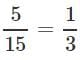QUESTION: 8

Look at the number line and choose the right option.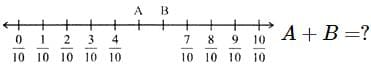Solution: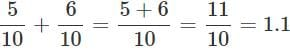QUESTION: 9

Compare: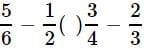Solution: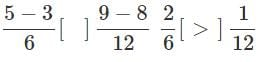QUESTION: 10

If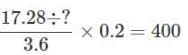then what should come in place of '?'

Solution:

we have,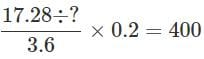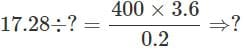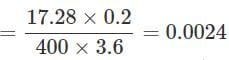Use Code STAYHOME200 and get INR 200 additional OFF Use Coupon Code